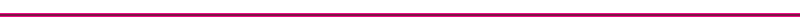Cylinder Heat Transfer Processes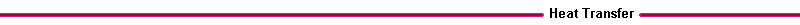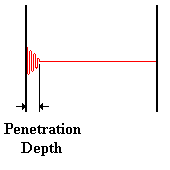Figure 14. Penetration Depth The heat transfer from the hot combustion gases is includes forced convection through the hot gas boundary layer, conduction through the cylinder wall, and forced convection (including boiling) into the fluid coolant in the head, engine block, and piston. There is a small ( about 5 %) radiative component of heat transfer from the gas to the cylinder walls. The heat transfer process is periodic due to the piston motion. However, the engine speed is usually high enough so that the temperature fluctuations only penetrate about a millimeter into the cylinder wall. We use the unsteady heat conduction equation to examine the effect of the periodic combustion on the temperature profile in the cylinder wall.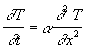where T is temperature C, t is time (sec), x is distance (m), and a is the thermal diffusivity (m2/s). Scale analysis of the above equation gives an approximate relation for the penetration depth x: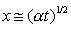An engine speed, w, of 1000 rpm, has a time scale t ofThe thermal diffusivity of steel is about a = 20 x 10 -6 m2/s, so the penetration depth is approximately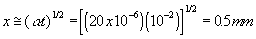Since the penetration depth is a very small fraction of the cylinder wall thickness, the cylinder wall can be assumed to have a temperature profile that is not changing in time. Cylinder Heat Flux and Temperatures The surface temperature of the cylinder can be measured with thermocouples, and the resulting cylinder heat flux deduced from Fourier's conduction equation and the unsteady heat conduction equation. The figure below is a representative graph of the cylinder heat flux as a function of crank angle.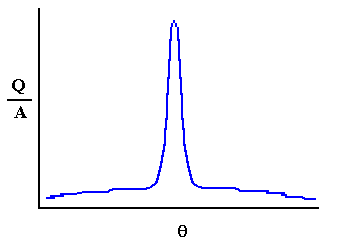Figure 15. Heat Flux versus Crank Angle The heat flux begins rising when the combustion flame impacts the cylinder wall, has a maximum at peak cylinder pressure when gas temperatures peak, typically 20 degrees after Top Dead Center (TDC). The peak heat fluxes are on the order of 1 to 3 MW/m2. The heat flux increases with increasing engine load and speed. As the cylinder wall temperature increases, the piston and ring friction will be reduced, decreasing the fuel consumption, the heat flux to the wall from the combustion gases will decrease, and the formulation of pollutants such as nitrous oxides also increases.